# AP Chemistry : Precipitates and Calculations

## Example Questions

### Example Question #1 : Precipitates And Calculations

A chemist combines 300 mL of a 0.3 M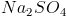solution with 200 mL of 0.4 M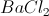solution. How many grams of precipitate form?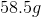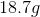No precipitate is formed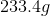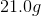Explanation:

First, let us write out an ion exchance reaction for the reactants: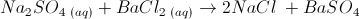By solubility rules,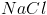is soluble in water and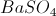is not. Our new reaction is: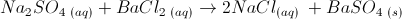Now we will calculate the theoretical yield of each reactant.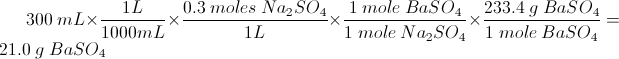Now we perform the same calculation beginning with: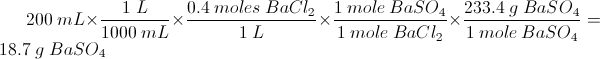The limiting reagent isand this reaction produces 18.7 g precipitate.

### Example Question #2 : Precipitates And Calculations

A chemist boils off the water from 234 mL of a 0.4 M solution of KCl. What is the mass of the remaining solid?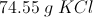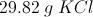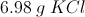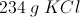Explanation: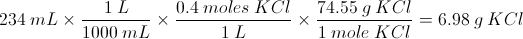### Example Question #3 : Precipitates And Calculations

A chemist boils off 344 mL of a 0.35 M solution of sodium hydroxide. How much solid remains?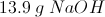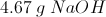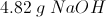No solid will remain as it will boil off with the waterExplanation:

First, you must recognize that the chemical formula for sodium hydroxide is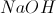. The mass of the boiled solution is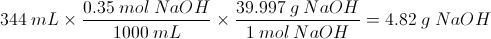### Example Question #31 : Solutions

What is the mass of the solid left over after boiling off 100mL of 0.4M NaCl solution?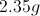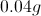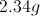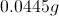Explanation:

The remaining mass with be equal to the mass of the sodium chloride in the solution. Once the solvent (water) evaporates, the solute will remain.

Atomic mass of sodium is ~23. Atomic mass of chlorine is ~35.5. Molecular mass of NaCl is ~58.5.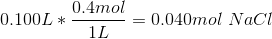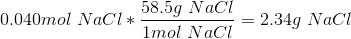### Example Question #5 : Precipitates And Calculations

A chemist has 5.2L of a 0.3M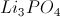solution. If the solvent were boiled off, what would be the mass of the remaining solid?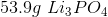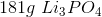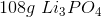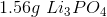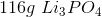Explanation:

First we will figure out the number of moles ofthat we have. We have 5.2 L of a 0.3 M solution, so: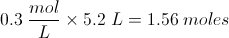Now the problem is to find the mass of 1.56 moles of. First, we need to know the molecular weight of. We will go to the periodic table and add up the mass of each element present.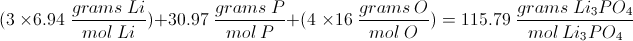Now the problem is simple as we have the molar mass and the number of desired moles.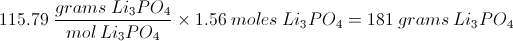### Example Question #1 : Precipitates And Calculations

Given a pKof 6.37 for the first deprotonation of carbonic acid (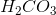), what is the ratio of bicarbonate (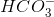) to carbonic acid () at pH 5.60?

Assume that the effect of the deprotonation of bicarbonate is negligible in your calculations.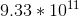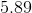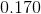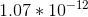Explanation:

Use the Henderson-Hasselbalch equation to determine the ratio of bicarbonate to carbonic acid in solution: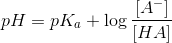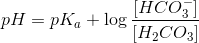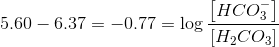Solve for the ratio we need to answer the question: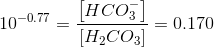### Example Question #7 : Precipitates And Calculations

The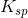of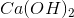(at 298K) is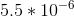. What is the molar solubility of the hydroxide ion (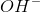) in a saturated solution of?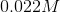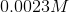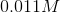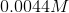Explanation:

The dissociation of calcium hydroxide in aqueous solution is: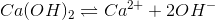Theof calcium hydroxide is related to the dissolved concentrations of its counterions: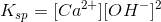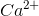andare produced in a molar ratio of 1:2; for each molecule of calcium hydroxide that dissolves: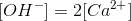Given avalue of, the molar solubilities of each counterion may be determined by setting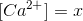. It follows that: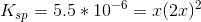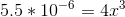Now, we can use basic algebra to solve for: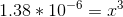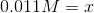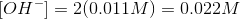Since we set, and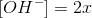, multiplying the value ofby two gives the correct answer, which is 0.022M.

### Example Question #1 : Precipitates And Calculations

What type of reaction is also known as a precipitation reaction?

Combination

Single replacement

Decomposition

Double replacement

Combustion